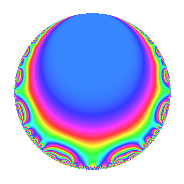# Properties

 Label 2015.1.bq.aLevel 2015 Weight 1 Character orbit 2015.bq Analytic conductor 1.006 Analytic rank 0 Dimension 2 Projective image $$D_{3}$$ CM disc. -155 Inner twists 4

# Related objects

## Newspace parameters

 Level: $$N$$ = $$2015 = 5 \cdot 13 \cdot 31$$ Weight: $$k$$ = $$1$$ Character orbit: $$[\chi]$$ = 2015.bq (of order $$6$$ and degree $$2$$)

## Newform invariants

 Self dual: No Analytic conductor: $$1.00561600046$$ Analytic rank: $$0$$ Dimension: $$2$$ Coefficient field: $$\Q(\zeta_{6})$$ Coefficient ring: $$\Z[a_1, \ldots, a_{4}]$$ Coefficient ring index: $$1$$ Projective image $$D_{3}$$ Projective field Galois closure of 3.1.26195.1 Artin image size $$18$$ Artin image $C_3\times S_3$ Artin field Galois closure of 6.0.629334875.1

## $q$-expansion

The $$q$$-expansion and trace form are shown below.

 $$f(q)$$ $$=$$ $$q$$ $$-2 \zeta_{6} q^{3}$$ $$+ \zeta_{6}^{2} q^{4}$$ $$+ q^{5}$$ $$+ 3 \zeta_{6}^{2} q^{9}$$ $$+O(q^{10})$$ $$q$$ $$-2 \zeta_{6} q^{3}$$ $$+ \zeta_{6}^{2} q^{4}$$ $$+ q^{5}$$ $$+ 3 \zeta_{6}^{2} q^{9}$$ $$+ 2 q^{12}$$ $$+ \zeta_{6}^{2} q^{13}$$ $$-2 \zeta_{6} q^{15}$$ $$-\zeta_{6} q^{16}$$ $$-\zeta_{6}^{2} q^{17}$$ $$-\zeta_{6}^{2} q^{19}$$ $$+ \zeta_{6}^{2} q^{20}$$ $$+ \zeta_{6} q^{23}$$ $$+ q^{25}$$ $$+ 4 q^{27}$$ $$+ q^{31}$$ $$-3 \zeta_{6} q^{36}$$ $$+ \zeta_{6} q^{37}$$ $$+ 2 q^{39}$$ $$+ \zeta_{6} q^{41}$$ $$-\zeta_{6}^{2} q^{43}$$ $$+ 3 \zeta_{6}^{2} q^{45}$$ $$+ 2 \zeta_{6}^{2} q^{48}$$ $$-\zeta_{6} q^{49}$$ $$-2 q^{51}$$ $$-\zeta_{6} q^{52}$$ $$- q^{53}$$ $$-2 q^{57}$$ $$-\zeta_{6}^{2} q^{59}$$ $$+ 2 q^{60}$$ $$+ q^{64}$$ $$+ \zeta_{6}^{2} q^{65}$$ $$+ \zeta_{6} q^{68}$$ $$-2 \zeta_{6}^{2} q^{69}$$ $$-\zeta_{6}^{2} q^{71}$$ $$+ 2 q^{73}$$ $$-2 \zeta_{6} q^{75}$$ $$+ \zeta_{6} q^{76}$$ $$-\zeta_{6} q^{80}$$ $$-5 \zeta_{6} q^{81}$$ $$- q^{83}$$ $$-\zeta_{6}^{2} q^{85}$$ $$- q^{92}$$ $$-2 \zeta_{6} q^{93}$$ $$-\zeta_{6}^{2} q^{95}$$ $$+O(q^{100})$$ $$\operatorname{Tr}(f)(q)$$ $$=$$ $$2q$$ $$\mathstrut -\mathstrut 2q^{3}$$ $$\mathstrut -\mathstrut q^{4}$$ $$\mathstrut +\mathstrut 2q^{5}$$ $$\mathstrut -\mathstrut 3q^{9}$$ $$\mathstrut +\mathstrut O(q^{10})$$ $$2q$$ $$\mathstrut -\mathstrut 2q^{3}$$ $$\mathstrut -\mathstrut q^{4}$$ $$\mathstrut +\mathstrut 2q^{5}$$ $$\mathstrut -\mathstrut 3q^{9}$$ $$\mathstrut +\mathstrut 4q^{12}$$ $$\mathstrut -\mathstrut q^{13}$$ $$\mathstrut -\mathstrut 2q^{15}$$ $$\mathstrut -\mathstrut q^{16}$$ $$\mathstrut +\mathstrut q^{17}$$ $$\mathstrut +\mathstrut q^{19}$$ $$\mathstrut -\mathstrut q^{20}$$ $$\mathstrut +\mathstrut q^{23}$$ $$\mathstrut +\mathstrut 2q^{25}$$ $$\mathstrut +\mathstrut 8q^{27}$$ $$\mathstrut +\mathstrut 2q^{31}$$ $$\mathstrut -\mathstrut 3q^{36}$$ $$\mathstrut +\mathstrut q^{37}$$ $$\mathstrut +\mathstrut 4q^{39}$$ $$\mathstrut +\mathstrut q^{41}$$ $$\mathstrut +\mathstrut q^{43}$$ $$\mathstrut -\mathstrut 3q^{45}$$ $$\mathstrut -\mathstrut 2q^{48}$$ $$\mathstrut -\mathstrut q^{49}$$ $$\mathstrut -\mathstrut 4q^{51}$$ $$\mathstrut -\mathstrut q^{52}$$ $$\mathstrut -\mathstrut 2q^{53}$$ $$\mathstrut -\mathstrut 4q^{57}$$ $$\mathstrut +\mathstrut q^{59}$$ $$\mathstrut +\mathstrut 4q^{60}$$ $$\mathstrut +\mathstrut 2q^{64}$$ $$\mathstrut -\mathstrut q^{65}$$ $$\mathstrut +\mathstrut q^{68}$$ $$\mathstrut +\mathstrut 2q^{69}$$ $$\mathstrut +\mathstrut q^{71}$$ $$\mathstrut +\mathstrut 4q^{73}$$ $$\mathstrut -\mathstrut 2q^{75}$$ $$\mathstrut +\mathstrut q^{76}$$ $$\mathstrut -\mathstrut q^{80}$$ $$\mathstrut -\mathstrut 5q^{81}$$ $$\mathstrut -\mathstrut 2q^{83}$$ $$\mathstrut +\mathstrut q^{85}$$ $$\mathstrut -\mathstrut 2q^{92}$$ $$\mathstrut -\mathstrut 2q^{93}$$ $$\mathstrut +\mathstrut q^{95}$$ $$\mathstrut +\mathstrut O(q^{100})$$

## Character Values

We give the values of $$\chi$$ on generators for $$\left(\mathbb{Z}/2015\mathbb{Z}\right)^\times$$.

 $$n$$ $$716$$ $$807$$ $$1861$$ $$\chi(n)$$ $$-1$$ $$-1$$ $$\zeta_{6}^{2}$$

## Embeddings

For each embedding $$\iota_m$$ of the coefficient field, the values $$\iota_m(a_n)$$ are shown below.

For more information on an embedded modular form you can click on its label.

Label $$\iota_m(\nu)$$ $$a_{2}$$ $$a_{3}$$ $$a_{4}$$ $$a_{5}$$ $$a_{6}$$ $$a_{7}$$ $$a_{8}$$ $$a_{9}$$ $$a_{10}$$
464.1
 0.5 − 0.866025i 0.5 + 0.866025i
0 −1.00000 + 1.73205i −0.500000 0.866025i 1.00000 0 0 0 −1.50000 2.59808i 0
1394.1 0 −1.00000 1.73205i −0.500000 + 0.866025i 1.00000 0 0 0 −1.50000 + 2.59808i 0
 $$n$$: e.g. 2-40 or 990-1000 Significant digits: Format: Complex embeddings Normalized embeddings Satake parameters Satake angles

## Inner twists

Char. orbit Parity Mult. Self Twist Proved
1.a Even 1 trivial yes
155.c Odd 1 CM by $$\Q(\sqrt{-155})$$ yes
13.c Even 1 yes
2015.bq Odd 1 yes

## Hecke kernels

This newform can be constructed as the intersection of the kernels of the following linear operators acting on $$S_{1}^{\mathrm{new}}(2015, [\chi])$$:

 $$T_{2}$$ $$T_{3}^{2}$$ $$\mathstrut +\mathstrut 2 T_{3}$$ $$\mathstrut +\mathstrut 4$$